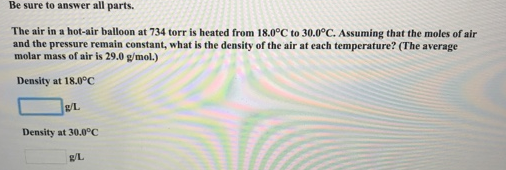# Problem: Be sure to answer all parts. The air in a hot-air balloon at 734 torr is heated from 18.00°C to 30.00°C, Assuming that the moles of air and the pressure remain constant, what is the density of the air at each temperature? (The average molar mass of air is 29.0 g/mol.)

###### FREE Expert Solution
81% (22 ratings)###### Problem Details

Be sure to answer all parts.

The air in a hot-air balloon at 734 torr is heated from 18.00°C to 30.00°C, Assuming that the moles of air and the pressure remain constant, what is the density of the air at each temperature? (The average molar mass of air is 29.0 g/mol.)